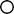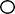# GATE 2021 Production and Industrial Engineering Previous Year PaperGATE 2021 Production and Industrial Engineering Previous Year Paper

General Aptitude (GA)

Q.1 – Q.5 Multiple Choice Question (MCQ), carry ONE mark each (for each wrong answer: – 1/3).

Q.6 – Q. 10 Multiple Choice Question (MCQ), carry TWO marks each (for each wrong answer: – 2/3).

Production & Industrial Engineering (PI)

1. – Q.17 Multiple Choice Question (MCQ), carry ONE mark each (for each wrong answer: – 1/3).Q.18 – Q.25 Numerical Answer Type (NAT), carry ONE mark each (no negative marks).

Q.26 – Q.36 Multiple Choice Question (MCQ), carry TWO mark each (for each wrong answer: – 2/3).

Q.37 – Q.55 Numerical Answer Type (NAT), carry TWO mark each (no negative marks).